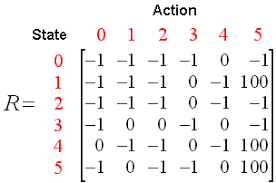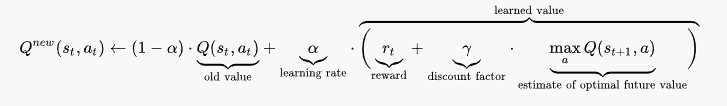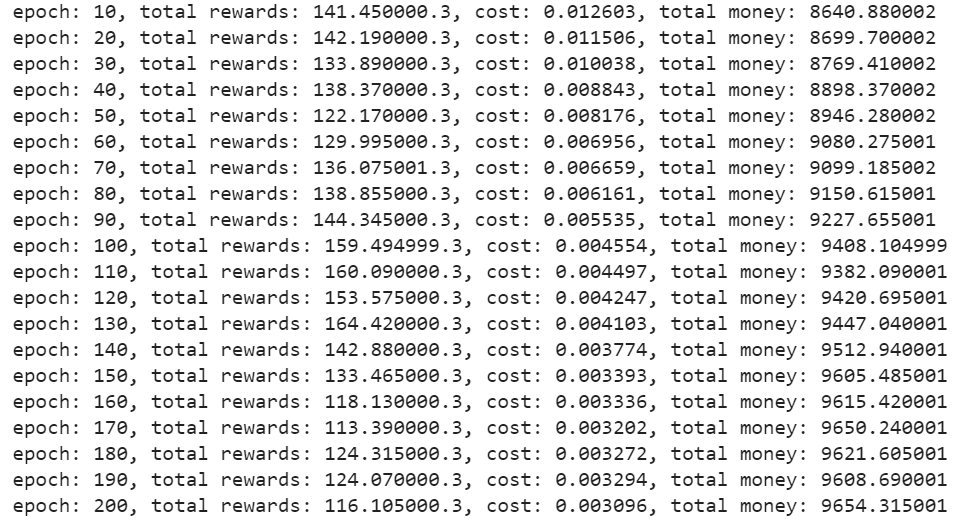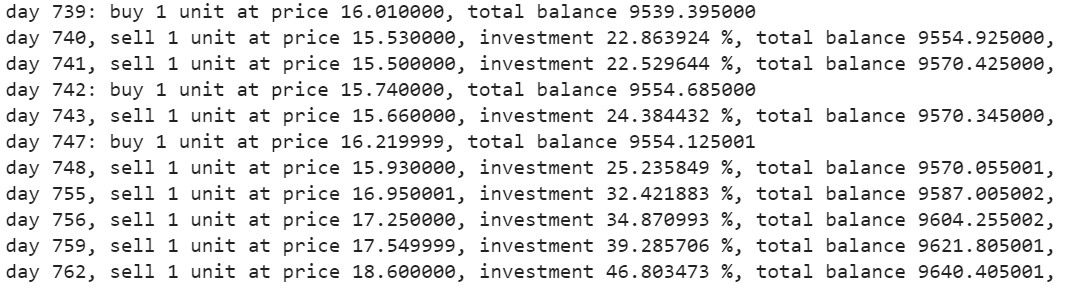Ekta Shah — Updated On January 20th, 2021

This article was published as a part of the Data Science Blogathon.

## Introduction

Bear and bull are terms that you will get to hear in the stock market often. A bear run is a term that suggests a decline in the market prices over a long time while a bull run refers to its opposite. These are terms used by traders who deal in intraday trading. Intraday trading is a form of speculation in securities in which a trader buys and sells a financial instrument within the same trading day, such that all market positions are closed before the market closes for that day. A large volume of financial instruments is traded via the Intra Day trading method.

This has been conventionally working with the trade plan and news trends. With the advent of Data Science and Machine Learning, various research approaches have been designed to automate this manual process. This automated trading process will help in giving suggestions at the right time with better calculations. An automated trading strategy that gives maximum profit is highly desirable for mutual funds and hedge funds. The kind of profitable returns that is expected will come with some amount of potential risk. Designing a profitable automated trading strategy is a complex task.

Every human being wants to earn to their maximum potential in the stock market. It is very important to design a balanced and low-risk strategy that can benefit most people. One such approach talks about using reinforcement learning agents to provide us with automated trading strategies based on the basis of historical data.

## Reinforcement Learning

Reinforcement learning is a type of machine learning where there are environments and agents. These agents take actions to maximize rewards. Reinforcement learning has a very huge potential when it is used for simulations for training an AI model. There is no label associated with any data, reinforcement learning can learn better with very few data points. All decisions, in this case, are taken sequentially. The best example would be found in Robotics and Gaming.## Q – Learning

Q-learning is a model-free reinforcement learning algorithm. It informs the agent what action to undertake according to the circumstances. It is a value-based method that is used to supply information to an agent for the impending action.  It is regarded as an off-policy algorithm as the q-learning function learns from actions that are outside the current policy, like taking random actions, and therefore a policy isn’t needed.

Q here stands for Quality. Quality refers to the action quality as to how beneficial that reward will be in accordance with the action taken. A Q-table is created with dimensions [state,action].An agent interacts with the environment in either of the two ways – exploit and explore. An exploit option suggests that all actions are considered and the one that gives maximum value to the environment is taken. An explore option is one where a random action is considered without considering the maximum future reward.Q of st and at is represented by a formula that calculates the maximum discounted future reward when an action is performed in a state s.

The defined function will provide us with the maximum reward at the end of the n number of training cycles or iterations.

Q-learning will rate each and every action and the one with the maximum value will be selected further. Q-Learning is based on learning the values from the Q-table. It functions well without the reward functions and state transition probabilities.

## Reinforcement Learning in Stock Trading

Reinforcement learning can solve various types of problems. Trading is a continuous task without any endpoint. Trading is also a partially observable Markov Decision Process as we do not have complete information about the traders in the market. Since we don’t know the reward function and transition probability, we use model-free reinforcement learning which is Q-Learning.

Steps to run an RL agent:

1. Install Libraries

2. Fetch the Data

3. Define the Q-Learning Agent

4. Train the Agent

5. Test the Agent

6. Plot the Signals

#### Install Libraries

Install and import the required NumPy, pandas, matplotlib, seaborn, and yahoo finance libraries.

```import numpy as np
import pandas as pd
import matplotlib.pyplot as plt
import seaborn as sns
sns.set()
from pandas_datareader import data as pdr
import fix_yahoo_finance as yf
from collections import deque
import random
Import tensorflow.compat.v1 as tf
tf.compat.v1.disable_eager_execution()```

#### Fetch the Data

Use the Yahoo Finance library to fetch the data for a particular stock. The stock used here for our analysis is Infosys stocks.

```yf.pdr_override()
df_full = pdr.get_data_yahoo("INFY", start="2018-01-01").reset_index()
df_full.to_csv(‘INFY.csv',index=False)

This code will create a data frame called df_full that will contain the stock prices of INFY over the course of 2 years.

#### Define the Q-Learning Agent

The first function is the Agent class defines the state size, window size, batch size, deque which is the memory used, inventory as a list. It also defines some static variables like epsilon, decay, gamma, etc. Two neural network layers are defined for the buy, hold, and sell call. The GradientDescentOptimizer is also used.

The Agent has functions defined for buy and sell options. The get_state and act function makes use of the Neural network for generating the next state of the neural network. The rewards are subsequently calculated by adding or subtracting the value generated by executing the call option. The action taken at the next state is influenced by the action taken on the previous state. 1 refers to a Buy call while 2 refers to a Sell call. In every iteration, the state is determined on the basis of which an action is taken which will either buy or sell some stocks. The overall rewards are stored in the total profit variable.

```df= df_full.copy()
name = 'Q-learning agent'
class Agent:
def __init__(self, state_size, window_size, trend, skip, batch_size):
self.state_size = state_size
self.window_size = window_size
self.half_window = window_size // 2
self.trend = trend
self.skip = skip
self.action_size = 3
self.batch_size = batch_size
self.memory = deque(maxlen = 1000)
self.inventory = []
self.gamma = 0.95
self.epsilon = 0.5
self.epsilon_min = 0.01
self.epsilon_decay = 0.999
tf.reset_default_graph()
self.sess = tf.InteractiveSession()
self.X = tf.placeholder(tf.float32, [None, self.state_size])
self.Y = tf.placeholder(tf.float32, [None, self.action_size])
feed = tf.layers.dense(self.X, 256, activation = tf.nn.relu)
self.logits = tf.layers.dense(feed, self.action_size)
self.cost = tf.reduce_mean(tf.square(self.Y - self.logits))
self.cost
)
self.sess.run(tf.global_variables_initializer())
def act(self, state):
if random.random() <= self.epsilon:
return random.randrange(self.action_size)
return np.argmax(
self.sess.run(self.logits, feed_dict = {self.X: state})
)
def get_state(self, t):
window_size = self.window_size + 1
d = t - window_size + 1
block = self.trend[d : t + 1] if d >= 0 else -d * [self.trend] + self.trend[0 : t + 1]
res = []
for i in range(window_size - 1):
res.append(block[i + 1] - block[i])
return np.array([res])
def replay(self, batch_size):
mini_batch = []
l = len(self.memory)
for i in range(l - batch_size, l):
mini_batch.append(self.memory[i])
replay_size = len(mini_batch)
X = np.empty((replay_size, self.state_size))
Y = np.empty((replay_size, self.action_size))
states = np.array([a for a in mini_batch])
new_states = np.array([a for a in mini_batch])
Q = self.sess.run(self.logits, feed_dict = {self.X: states})
Q_new = self.sess.run(self.logits, feed_dict = {self.X: new_states})
for i in range(len(mini_batch)):
state, action, reward, next_state, done = mini_batch[i]
target = Q[i]
target[action] = reward
if not done:
target[action] += self.gamma * np.amax(Q_new[i])
X[i] = state
Y[i] = target
cost, _ = self.sess.run(
[self.cost, self.optimizer], feed_dict = {self.X: X, self.Y: Y}
)
if self.epsilon > self.epsilon_min:
self.epsilon *= self.epsilon_decay
return cost
starting_money = initial_money
states_sell = []
inventory = []
state = self.get_state(0)
for t in range(0, len(self.trend) - 1, self.skip):
action = self.act(state)
next_state = self.get_state(t + 1)
if action == 1 and initial_money >= self.trend[t] and t < (len(self.trend) - self.half_window):
inventory.append(self.trend[t])
initial_money -= self.trend[t]
print('day %d: buy 1 unit at price %f, total balance %f'% (t, self.trend[t], initial_money))
elif action == 2 and len(inventory):
bought_price = inventory.pop(0)
initial_money += self.trend[t]
states_sell.append(t)
try:
invest = ((close[t] - bought_price) / bought_price) * 100
except:
invest = 0
print(
'day %d, sell 1 unit at price %f, investment %f %%, total balance %f,'
% (t, close[t], invest, initial_money)
)
state = next_state
invest = ((initial_money - starting_money) / starting_money) * 100
total_gains = initial_money - starting_money
def train(self, iterations, checkpoint, initial_money):
for i in range(iterations):
total_profit = 0
inventory = []
state = self.get_state(0)
starting_money = initial_money
for t in range(0, len(self.trend) - 1, self.skip):
action = self.act(state)
next_state = self.get_state(t + 1)
if action == 1 and starting_money >= self.trend[t] and t < (len(self.trend) - self.half_window):
inventory.append(self.trend[t])
starting_money -= self.trend[t]
elif action == 2 and len(inventory) > 0:
bought_price = inventory.pop(0)
total_profit += self.trend[t] - bought_price
starting_money += self.trend[t]
invest = ((starting_money - initial_money) / initial_money)
self.memory.append((state, action, invest,
next_state, starting_money < initial_money))
state = next_state
batch_size = min(self.batch_size, len(self.memory))
cost = self.replay(batch_size)
if (i+1) % checkpoint == 0:
print('epoch: %d, total rewards: %f.3, cost: %f, total money: %f'%(i + 1, total_profit, cost,
starting_money))```

#### Train the Agent

Once the agent is defined, initialize the agent. Specify the number of iterations, initial money, etc to train the agent to decide the buy or sell options.

```close = df.Close.values.tolist()
initial_money = 10000
window_size = 30
skip = 1
batch_size = 32
agent = Agent(state_size = window_size,
window_size = window_size,
trend = close,
skip = skip,
batch_size = batch_size)
agent.train(iterations = 200, checkpoint = 10, initial_money = initial_money)```

Output –#### Test the Agent

The buy function will return the buy, sell, profit, and investment figures.

`states_buy, states_sell, total_gains, invest = agent.buy(initial_money = initial_money)`

#### Plot the calls

Plot the total gains vs the invested figures. All buy and sell calls have been appropriately marked according to the buy/sell options as suggested by the neural network.

```fig = plt.figure(figsize = (15,5))
plt.plot(close, color='r', lw=2.)
plt.plot(close, 'v', markersize=10, color='k', label = 'selling signal', markevery = states_sell)
plt.title('total gains %f, total investment %f%%'%(total_gains, invest))
plt.legend()
plt.savefig(name+'.png')
plt.show()```

Output –## End Notes

Q-Learning is such a technique that helps you develop an automated trading strategy. It can be used to experiment with the buy or sell options. There are a lot more Reinforcement Learning trading agents that can be experimented with. Try playing around with the different kinds of RL agents with different stocks.

The media shown in this article are not owned by Analytics Vidhya and is used at the Author’s discretion.### Our Top Authors

•## 8 thoughts on "Bear run or bull run, Can Reinforcement Learning help in Automated trading?"###### Manny says:January 16, 2021 at 10:08 pm
Just an FYI: I copied the code, ran it, and I am experimenting with it now. Just thought you would like to know, I got the following warnings. Thanks much. Many [*********************100%***********************] 1 of 1 completed WARNING:tensorflow:From E:\Stock Market Trading\Download Stock Prices\Bear_Bull Stock Market Automated Trading.py:64: dense (from tensorflow.python.keras.legacy_tf_layers.core) is deprecated and will be removed in a future version. Instructions for updating: Use keras.layers.Dense instead. WARNING:tensorflow:From C:\Users\Manny\anaconda3\lib\site-packages\tensorflow\python\keras\legacy_tf_layers\core.py:187: Layer.apply (from tensorflow.python.keras.engine.base_layer_v1) is deprecated and will be removed in a future version. Instructions for updating: Please use `layer.__call__` method instead. 2021-01-16 11:33:45.248164: I tensorflow/core/platform/cpu_feature_guard.cc:142] This TensorFlow binary is optimized with oneAPI Deep Neural Network Library (oneDNN)to use the following CPU instructions in performance-critical operations: AVX AVX2 To enable them in other operations, rebuild TensorFlow with the appropriate compiler flags. Reply###### Zoya says:November 10, 2021 at 12:14 am###### zoya says:May 25, 2022 at 11:37 pm
Why gain and investment is negative?? Reply###### Peter Jameson says:October 27, 2022 at 6:21 pm
An excellent presentation! How does the Reinforcement Learning algorithm compare to the naive model? That is, if we do nothing, just buy and hold the stock? If Reinforcement Learning does not improve on the simple buy and hold strategy then is it of any use? If we are interested in what to do tomorrow how does the Reinforcement Learning algorithm tell us what to do for tomorrow? Reply###### Dario says:December 14, 2022 at 7:54 pm